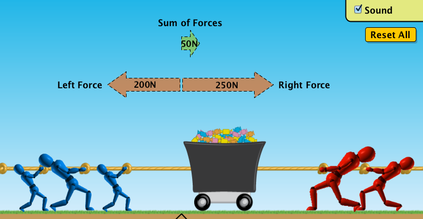Net Force
3 years ago
mrsjtcramer
Save
Edit
Host a game
Live GameLive
Homework
Solo Practice
Practice13 QuestionsShow answers
• Question 1
30 secondsQ. What is the net force?
5 N
45 N to the left
5 N to the right
45 N to the right
• Question 2
30 seconds
Q. What is a force?
the PUSH or PULL on an object
The MOTION an object creates
The objects HITTING one another
Going REALLY FAST
• Question 3
30 secondsQ. Which one is balanced?
top
bottom
both
• Question 4
30 secondsQ. Is it BALANCED?
Yes
No
• Question 5
30 secondsQ. What is the FORCE?
5 N
10 N
400 N
0 N
• Question 6
30 seconds
Q. What is the unit for FORCE?
N (Newtons)
lbs (pounds)
g (grams)
m/s (meters per second)
• Question 7
30 seconds
Q. If there are two forces going in the SAME direction, you would ...?
(-) Subtract the forces
Neither
(x) Multiply
• Question 8
60 secondsQ. Forces that have a force of Zero (0).
Balanced force
Unbalanced force
• Question 9
30 secondsQ. The FORCE that PULLS things down or back to EARTH.
Speed
Gravity
• Question 10
30 seconds
Q. If the net force is 0 the object will ...
CHANGES motion
STAY the SAME, NOT Move
• Question 11
60 secondsQ. This is an example of what kind of force?
Balanced Force
Pushing Force
Gravity Force
Unbalanced Force
• Question 12
30 seconds
Q. If you have balanced forces on a ball what happens
It STAYS the SAME
CHANGES its MOTION
:(
:)
• Question 13
900 secondsQ. What is the net force?